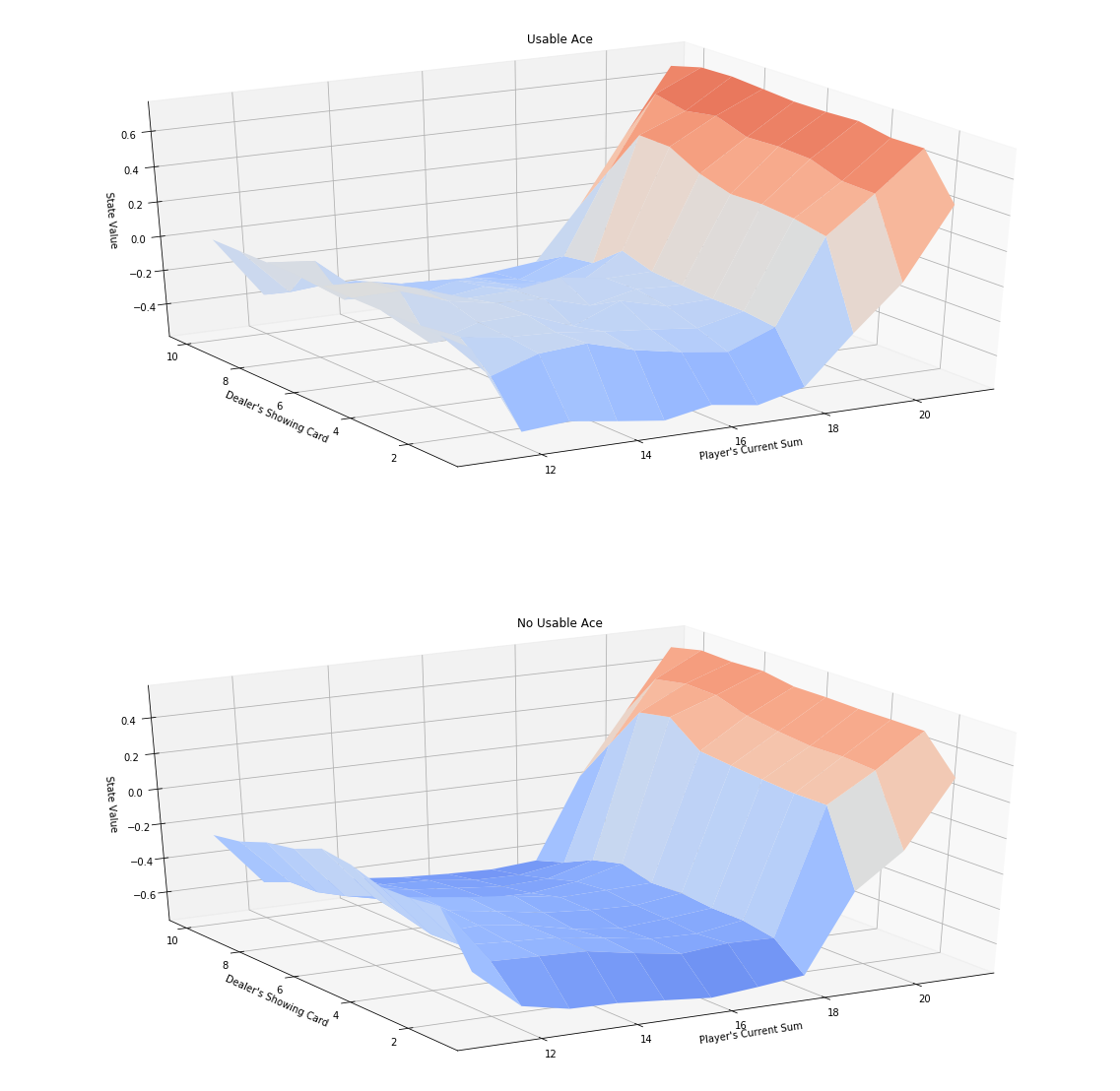# Monte Carlo Methods - Prediction¶

### 5.1. Blackgame Problem¶

In :
import sys
import gym
import numpy as np
from collections import defaultdict

from plot_utils import plot_blackjack_values, plot_policy


Use the code cell below to create an instance of the Blackjack environment.

In :
env = gym.make('Blackjack-v0')


Each state is a 3-tuple of:

• the player's current sum $\in \{0, 1, \ldots, 31\}$,
• the dealer's face up card $\in \{1, \ldots, 10\}$, and
• whether or not the player has a usable ace (no $=0$, yes $=1$).

The agent has two potential actions:

    STICK = 0
HIT = 1
In :
print(env.observation_space)
print(env.action_space)

Tuple(Discrete(32), Discrete(11), Discrete(2))
Discrete(2)

In :
env.action_space.sample()

Out:
1
In :
env.step(1)

Out:
((23, 2, False), -1, True, {})

For the below sample:

• The action was 1 (hit)
• The resulting state was (23, 2, False), -1, True.

Meaning that our sum was 23, the dealer has a visible 2, we don't have an usable ace.
Since we are over 21, we lost the game and the reward is -1; and the game is finished (True).

### Evaluate the random policy¶

The following code runs N episodes and show how state, actions and reward are obtained from the OpenAI environment

In :
N = 3
for i_episode in range(N):
state = env.reset()
while True:
print(state)
action = env.action_space.sample()
state, reward, done, info = env.step(action)
if done:
print('End game! Reward: ', reward)
print('You won :)\n') if reward > 0 else print('You lost :(\n')
break

(20, 3, False)
End game! Reward:  1.0
You won :)

(13, 1, True)
End game! Reward:  -1.0
You lost :(

(20, 1, False)
End game! Reward:  -1
You lost :(



### Evaluate a given policy¶

Let's evaluate the following policy:

The player almost always sticks if the sum of her cards exceeds 18. In particular, she selects action STICK with 80% probability if the sum is greater than 18; and, if the sum is 18 or below, she selects action HIT with 80% probability. The function generate_episode_from_limit_stochastic samples an episode using this policy.

The function accepts as input:

• bj_env: This is an instance of OpenAI Gym's Blackjack environment.

It returns as output:

• episode: This is a list of (state, action, reward) tuples (of tuples) and corresponds to $(S_0, A_0, R_1, \ldots, S_{T-1}, A_{T-1}, R_{T})$, where $T$ is the final time step. In particular, episode[i] returns $(S_i, A_i, R_{i+1})$, and episode[i], episode[i], and episode[i] return $S_i$, $A_i$, and $R_{i+1}$, respectively.
In :
def generate_episode_from_limit_stochastic(bj_env):
episode = []
state = bj_env.reset()
while True:
probs = [0.8, 0.2] if state > 18 else [0.2, 0.8]
action = np.random.choice(np.arange(2), p=probs)
next_state, reward, done, info = bj_env.step(action)
episode.append((state, action, reward))
state = next_state
if done:
break
return episode


The algorithm has three arguments:

• env: This is an instance of an OpenAI Gym environment.
• num_episodes: This is the number of episodes that are generated through agent-environment interaction.
• generate_episode: This is a function that returns an episode of interaction.
• gamma: This is the discount rate. It must be a value between 0 and 1, inclusive (default value: 1).

The algorithm returns as output the Q-Table:

• Q: This is a dictionary (of one-dimensional arrays) where Q[s][a] is the estimated action value corresponding to state s and action a.
In :
def mc_prediction_q(env, num_episodes, generate_episode, gamma=1.0):

# Initialize to zero the Total (summed) Return for each state-action
returns_sum = defaultdict(lambda: np.zeros(env.action_space.n))

# Initialize to zero the counter for every visit to each cell in the Q-table
N = defaultdict(lambda: np.zeros(env.action_space.n))

# Initialize to zero the value for every cell in the Q-table
Q = defaultdict(lambda: np.zeros(env.action_space.n))

# For each of the episodes run
for i_episode in range(1, num_episodes+1):

if i_episode % 1000 == 0:
print("\rEpisode {}/{}.".format(i_episode, num_episodes), end="")
sys.stdout.flush()

# Run the episode of the MDP and extract the values
episode = generate_episode(env)
states, actions, rewards = zip(*episode)

# Create the vector of discounts to be applied to the reward of each state
discounts = np.array([gamma**i for i in range(len(rewards)+1)])

# Update the values
for i, state in enumerate(states):

# R(s,a) = SUM(discounted rewards)
returns_sum[state][actions[i]] += sum(rewards[i:]*discounts[:-(1+i)])

# Increment the counter of visited states for that pair, N(s,a)
N[state][actions[i]] += 1.0

# Calculate the Q(s,a) value given the total Return R(s,a) and the times visited N(s,a)
Q[state][actions[i]] = returns_sum[state][actions[i]] / N[state][actions[i]]

return Q


### Run episodes and calculate $Q$-Table¶

we run our defined policy for N episodes and keep updating iteratively the values of the table when the pairs (s,a)are visited. The corresponding state-values are plotted

In :
# Obtain the action-value function
Q = mc_prediction_q(env, 500000, generate_episode_from_limit_stochastic)

# Obtain-back the corresponding state-value function
V_to_plot = dict((k,(k>18)*(np.dot([0.8, 0.2],v)) + (k<=18)*(np.dot([0.2, 0.8],v))) \
for k, v in Q.items())

# Plot the state-value function
plot_blackjack_values(V_to_plot)

Episode 500000/500000.